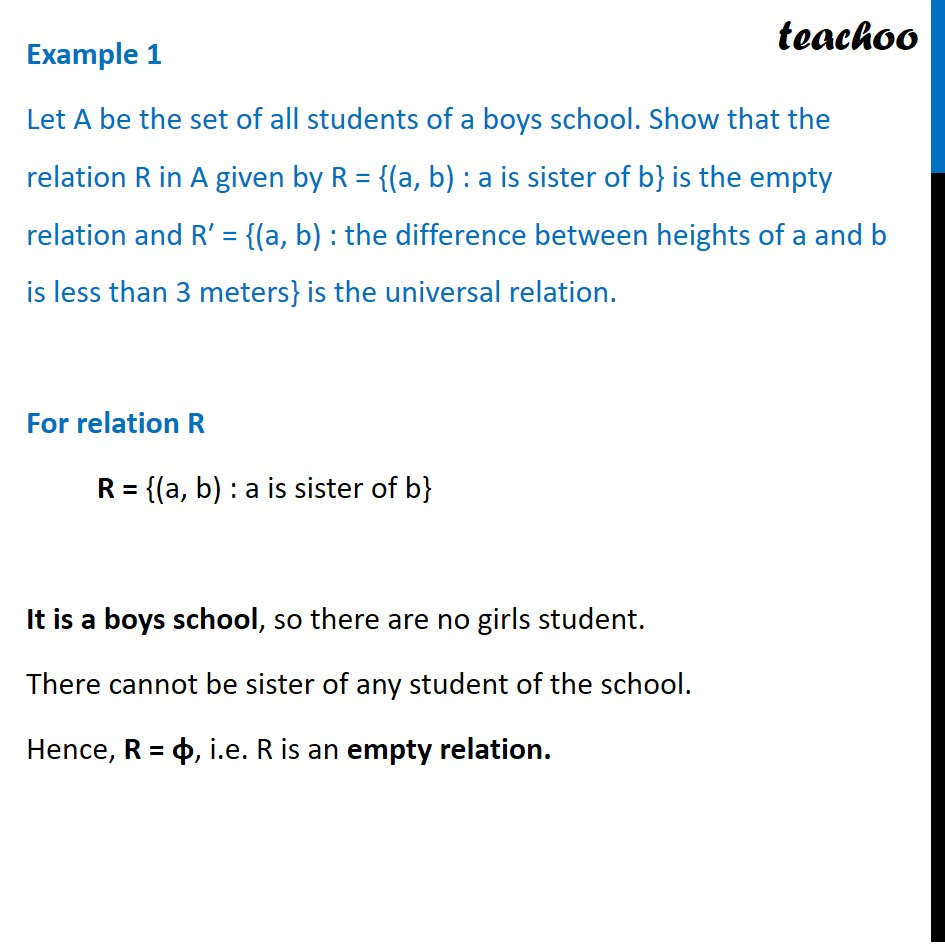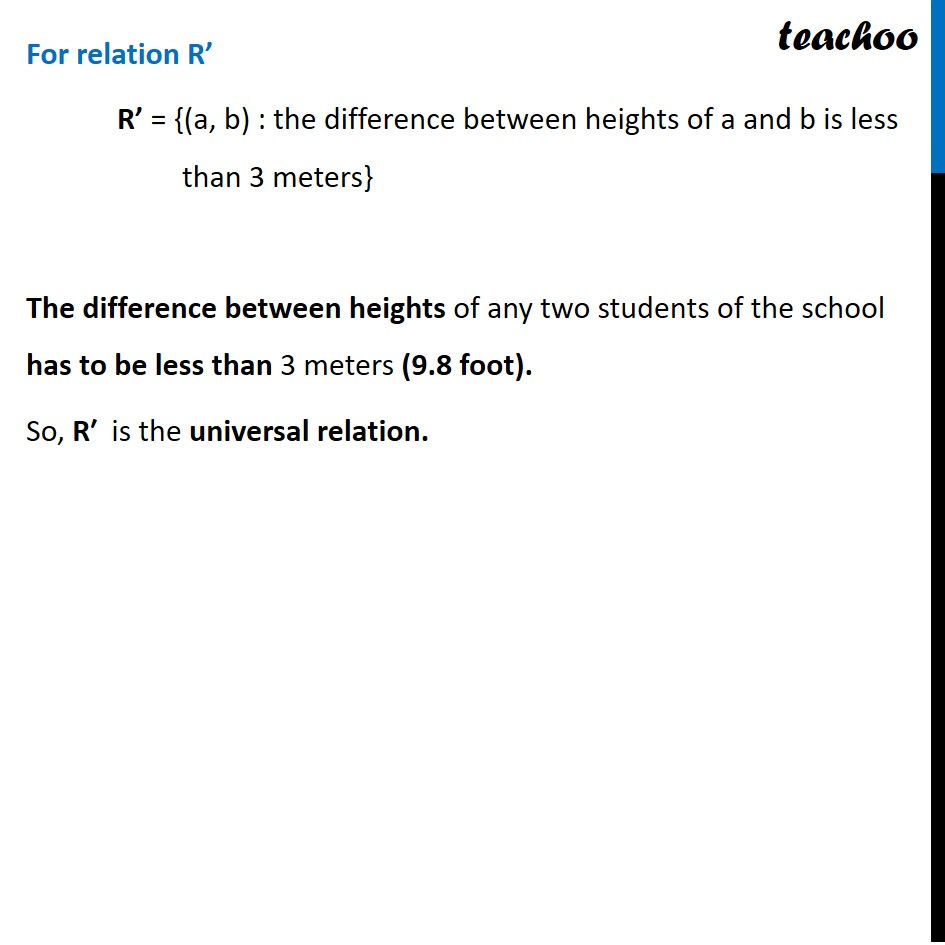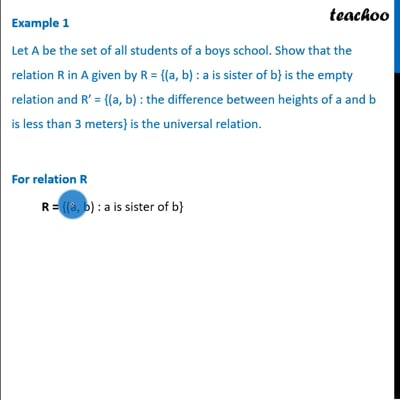Examples

Chapter 1 Class 12 Relation and Functions
Serial order wiseThis video is only available for Teachoo black users

Introducing your new favourite teacher - Teachoo Black, at only ₹83 per month

### Transcript

Example 1 Let A be the set of all students of a boys school. Show that the relation R in A given by R = {(a, b) : a is sister of b} is the empty relation and R′ = {(a, b) : the difference between heights of a and b is less than 3 meters} is the universal relation. For relation R R = {(a, b) : a is sister of b} It is a boys school, so there are no girls student. There cannot be sister of any student of the school. Hence, R = φ, i.e. R is an empty relation. For relation R’ R’ = {(a, b) : the difference between heights of a and b is less than 3 meters} The difference between heights of any two students of the school has to be less than 3 meters (9.8 foot). So, R′ is the universal relation.